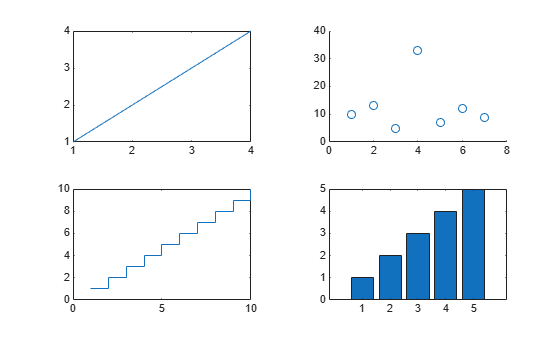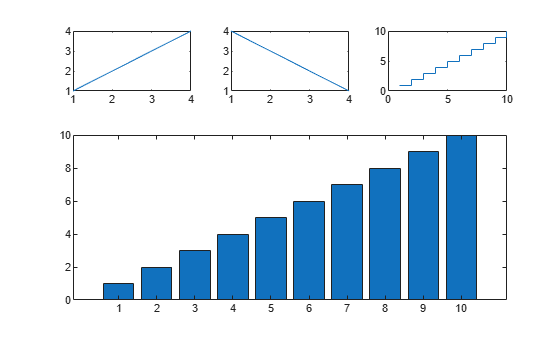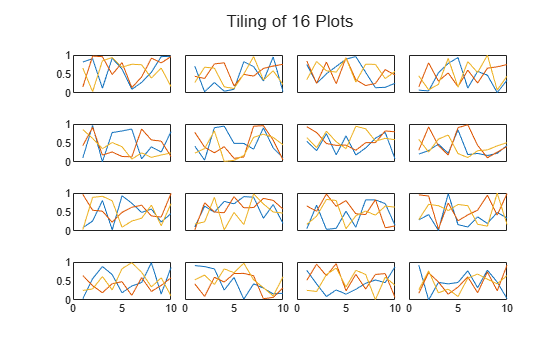# tilerowcol

Row and column numbers in tiled chart layout

Since R2022b

## Syntax

``[row,col] = tilerowcol(t,tnum)``
``[row,col] = tilerowcol(obj)``

## Description

example

````[row,col] = tilerowcol(t,tnum)` returns the row and column numbers for the specified tile number in the tiled chart layout `t`. Specify `tnum` as a scalar tile number or an array of tile numbers.This function does not support layouts that use the `"flow"`, `"vertical"`, or `"horizontal"` tile arrangements.```

example

````[row,col] = tilerowcol(obj)` returns the row and column numbers of the specified object in a tiled chart layout. For example, you can use this syntax to get the rows and columns for a specified axes object.```

## Examples

collapse all

Create a 2-by-2 tiled chart layout containing four plots.

```t = tiledlayout(2,2); nexttile plot([1 2 3 4]) nexttile scatter(1:7,[10 13 5 33 7 12 9]) nexttile stairs(1:10) nexttile bar(1:5)```Get the row and column numbers for the third tile.

`[row,col] = tilerowcol(t,3)`
```row = 2 ```
```col = 1 ```

Get the row and column numbers for all the tiles.

`[row,col] = tilerowcol(t,[1 2 3 4])`
```row = 1×4 1 1 2 2 ```
```col = 1×4 1 2 1 2 ```

Create a 2-by-2 tiled chart layout containing four plots.

```t = tiledlayout(2,2); nexttile plot([1 2 3 4]) nexttile scatter(1:7,[10 13 5 33 7 12 9]) nexttile stairs(1:10) nexttile bar(1:5)```The `Children` property of the `TiledChartLayout` object contains all the top-level objects in the layout. In this case, the top-level objects are axes. Note that the objects in the `Children` property are in the opposite order of their creation. To get the rows and columns in the order of creation, call the `flipud` function and pass the reordered array to the `tilerowcol` function.

`[row,col] = tilerowcol(flipud(t.Children))`
```row = 4×1 1 1 2 2 ```
```col = 4×1 1 2 1 2 ```

Create a 3-by-3 tiled chart layout containing three plots in the first row. Then create an axes object that spans across the remaining empty tiles (a 2-by-3 region). Create a bar chart in the spanned axes.

```tiledlayout(3,3) nexttile plot([1 2 3 4]) nexttile plot([4 3 2 1]) nexttile stairs(1:10) % Create spanned axes and bar chart spax = nexttile([2 3]); bar(spax,1:10)```Get the row and column numbers for the spanned axes. `tilerowcol` returns the numbers of the row and column at the upper-left corner of the spanned axes, not the entire set of spanned rows and columns.

`[r,c] = tilerowcol(spax)`
```r = 2 ```
```c = 1 ```

You can use the `tilerowcol` function to customize the axes in a specific row or column of a tiled chart layout. In this example, show the y-axis labels in the first column only, and show the x-axis labels in the bottom row only.

Create a 4-by-4 tiled chart layout containing 16 plots. Pass the `Children` property of the `TiledChartLayout` object to the `tilerowcol` function to get the rows and columns of all the tiles. Remove the y-axis tick labels from all the axes in the columns that have an index greater than 1. Remove the x-axis tick labels from all the axes that are not in the last row. Then add an overall title to the layout.

```t = tiledlayout(4,4,TileSpacing="compact"); for i=1:16 nexttile plot(rand(10,3)) end % Remove select y- and x-axis tick labels [row,col] = tilerowcol(t.Children); yticklabels(t.Children(col>1),"") xticklabels(t.Children(row<t.GridSize(1)),"") title(t,"Tiling of 16 Plots")```## Input Arguments

collapse all

Tiled chart layout containing the tiles you want to locate. Use the `tiledlayout` function to create the `TiledChartLayout` object.

The `TileArrangement` property of the `TiledChartLayout` object must be set to `"fixed"`, which is the default value when you call `tiledlayout(m,n)` to create a fixed number of tiles.

Tile numbers, specified as a scalar, vector, or array of positive integer values. If you specify a number that does not correspond to a tile number, `tilerowcol` returns `NaN` for that number.

Graphics object or an array of graphics objects that are children of a tiled chart layout. The types of objects you can specify include:

• Axes, such as `Axes`, `PolarAxes`, or `GeographicAxes` objects

• Standalone visualizations, such as `heatmap` charts

• Legends

• Colorbars

• `TiledChartLayout` objects that are nested within the tiles of a tiled chart layout

If a specified object is in one of the `"north"`, `"south"`, `"east"`, or `"west"` tiles, `tilenum` returns `NaN` for that object.

## Output Arguments

collapse all

Row and column numbers of the tiled chart layout, returned as two scalars, two vectors, two or two arrays containing the row and column indices of the tiled chart layout. If you query multiple tile numbers (or multiple objects) by specifying a vector or an array, `tilerowcol` returns the output as vectors or arrays of the same size.

`tilerowcol` returns `NaN` values if `tnum` does not correspond to a tile number, or if `obj` is a graphics objects in one of the `"north"`, `"south"`, `"east"`, or `"west"` tiles.

## Version History

Introduced in R2022b